# Inputs

## 1. Introduction

The total number of inputs is equal to 7.

Among these inputs, 1 is a standard input and 6 are advanced inputs.

Note: The standard input corresponds to a table to be filled with a set of working point described as indicated in the following section.

## 2.1 I-Ψ-N description

The “ I- Ψ-N description ” allows to define several working points.

The description must be defined by clicking on the button “Set values”.

Then, two ways are possible to fill in the table: either filling the table line per line or by importing an Excel file in which all the working points to be considered are defined.

Each working point is described by the following data:

• The line current, or current density (rms value)
• The control angle
• The speed
• The magnet temperature
Note:
1. There must be at least two working points.
2. The speed column must contain increasing speeds.
1. Line current

There are two common ways to define the electrical current.

The rms value of the line current supplied to the machine: “ Line current, rms” ( Line current, rms value ) must be provided.

2. Control angle

Considering the vector diagram shown below, the “ Control angle ” is the angle between the electromotive force E and the electrical current (J) (Ψ = (E, J)). It is an electrical angle. It must be set in a range of -90 to 90 degrees.

This range of values covers all the possible working point in motor convention.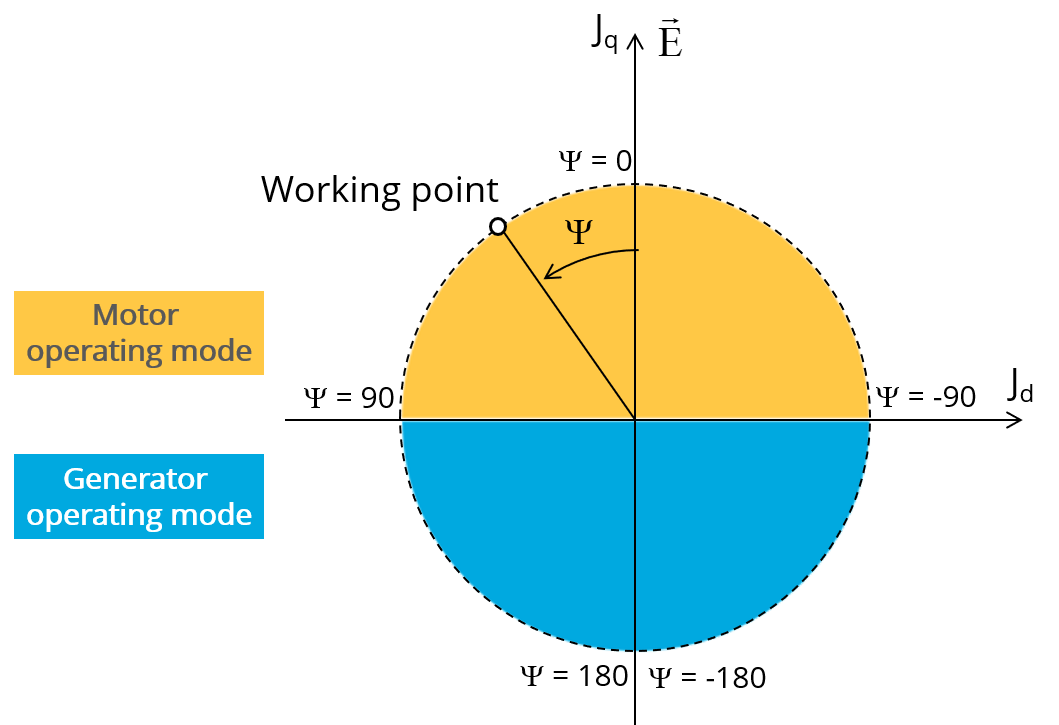Definition of the control angle Ψ - Motor convention
Note: The number of parallel paths and the winding connection are automatically considered in the results.
3. Speed

The imposed “ Speed ” ( Speed ) of the machine must be set. The speed column must contain increasing speeds.

4. Magnet temperature

The magnet temperature which is expected for each considered worked point must be set.

5. Process to define the list of working points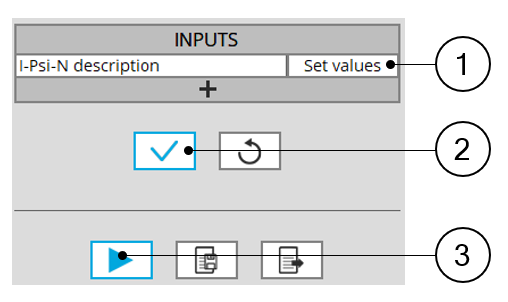List of working points to be defined 1 Click on the button “Set values” of the field “ I, Ψ, N description” to define the working points in a dialog box. Refer to the next illustration which shows how to fill the working point table. 2 Button to validate the user input data 3 Button to run the computations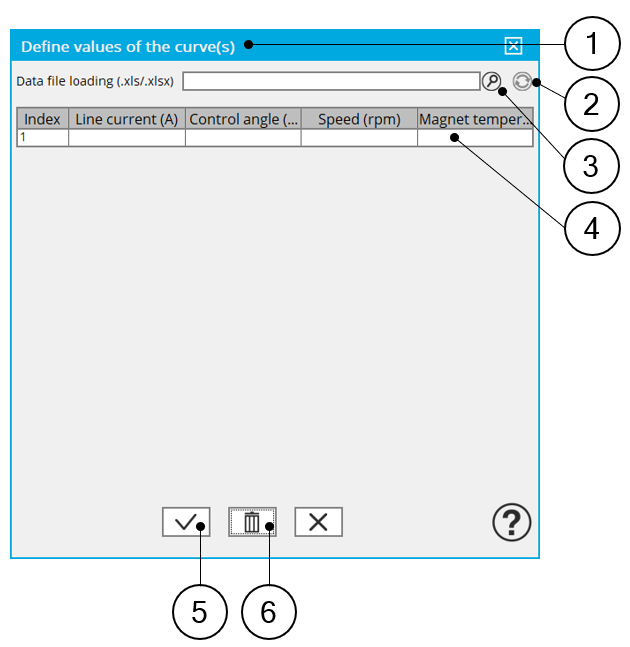Dialog box to define the list of working points 1 Dialog box opened after having clicked on the button “Set values” in the field “Cycle description” 2 Browse the folder to select an Excel file which is described the duty cycle 3 Button to refresh the table data when the considered Excel file has been modified 4 Fields to be filled with data to describe the duty cycle to be considered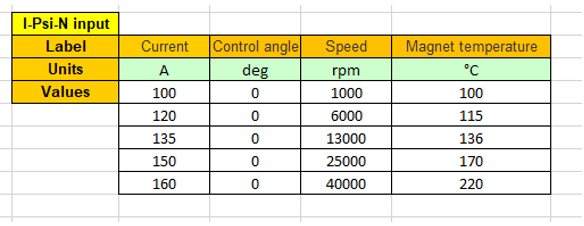Excel file template to define the list of working points

## 3.1 Maximum engine order / No. Points per electrical period

Two kinds of inputs are possible:

Define the Max. engine order ( Maximum engine order ) or the No. points / elec. Period ( Number of points per electric period ).

When decomposing the Maxwell pressure, applied on the stator, to get its harmonic contributions, the “ max. engine order ” ( Maximum engine order ) is required to compute its decomposition in function of the time.

At a practical point of view, when the maximum engine order is equal to N, that leads to consider 2*N computation points over a complete rotation period of the rotor.

Note:
1. The input "Engine order" is in connection with the frequency of vibration.

"Engine order" refers to a mechanical revolution period of the motor when frequency refers to the considered electrical period.

Obviously, both are linked with speed.

For instance, radiated sound power can be displayed either by considering frequency or engine order.

2. There are two possibilities, either set an engine order or a number of points per electrical period.

For transient computations the minimum needed number of points per electrical period is 40.

So, when the engine order is not high enough to reach this constraint It is automatically modified to get 40 computation points per electrical period.

## 3.2 Maximum mode / spatial order

The “ max. mode / spatial order ” ( Maximum mode / spatial order ) input allows the user to define the number of modes to be considered for the acoustic structural analysis. If the user selects 25, it means that the highest number of lobes in the stator deformation will be equal to 25 lobes. All deformations corresponding to more than 25 lobes will be dismissed.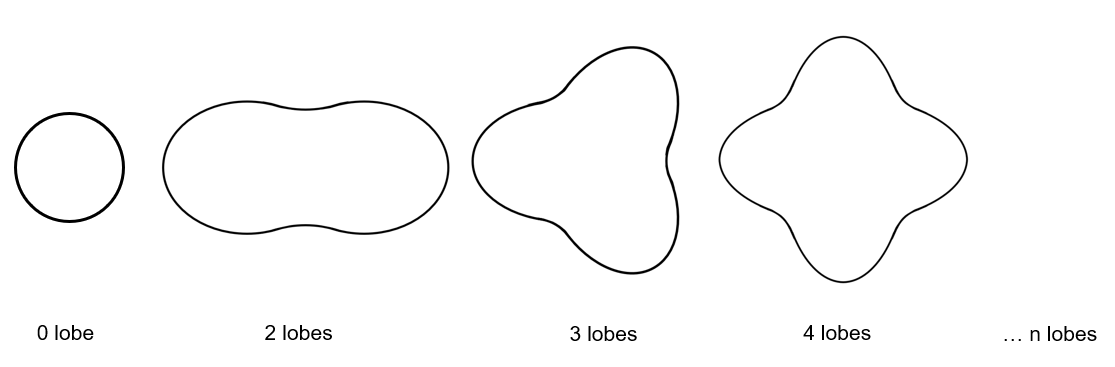Number of lobes of the stator mechanical structure

## 3.3 Number of computations per tooth pitch

The “ No. comp./tooth pitch ” ( Number of computations per tooth pitch ) allows to choose the number of Maxwell pressure evaluations per tooth. The more points selected, the more accurate the Maxwell pressure harmonic decomposition will be.

## 3.4 Number of points for speed interpolation

The “ No. points for speed interpolation ” (Number of points for speed interpolation) allows to manage the computation of the radiated sound power per engine order. It allows to manage the data interpolation between the speeds indicated as inputs. Thanks to that, the curves “Radiated sound power per engine order versus speed” and “weighted radiated sound power versus speed” can have a better discretization which leads to a better displaying of the local peaks.

The default value is equal to 100. The range of possible values is [50,300]

## 3.5 Mesh order

To get the results, the original computation is performed using a Finite Element Modeling.

Two levels of meshing can be considered for this finite element calculation: first order and second order.

This parameter influences the accuracy of results and the computation time.

By default, second order mesh is used.

## 3.6 Airgap mesh coefficient

The advanced user input “ Airgap mesh coefficient ” is a coefficient which adjusts the size of mesh elements inside the airgap. When the value of “ Airgap mesh coefficient ” decreases, the mesh elements get smaller, leading to a higher mesh density inside the airgap, increasing the computation accuracy.

The imposed Mesh Point (size of mesh elements touching points of the geometry) is described with the following parameters:

MeshPoint = (airgap) x (airgap mesh coefficient)

Airgap mesh coefficient is set to 1.5 by default.

The variation range of values for this parameter is [0.05; 2].

0.05 giving a very high mesh density and 2 giving a very coarse mesh density.

CAUTION:
Be aware, a very high mesh density does not always mean a better result quality.

However, this always leads to a huge number of nodes in the corresponding finite element model. So, it means a need of huge numerical memory and increases the computation time considerably.